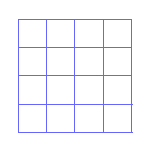Grade 6 maths word problems with answers are presented. Some of these problems are challenging and need more time to solve. Also detailed solutions and full explanations are included.

1. Two numbers N and 16 have LCM = 48 and GCF = 8. Find N.

2. If the area of a circle is 81pi square feet, find its circumference.

3. Find the greatest common factor of 24, 40 and 60.

4. In a given school, there are 240 boys and 260 girls.
a) What is the ratio of the number of girls to the number of boys?
b) What is the ratio of the number of boys to the total number of pupils in the school?

5. If Tim had lunch at \$50.50 and he gave 20% tip, how much did he spend?

6. Find k if 64 ÷ k = 4.

7. Little John had \$8.50. He spent \$1.25 on sweets and gave to his two friends \$1.20 each. How much money was left?

8. What is x if x + 2y = 10 and y = 3?

9. A telephone company charges initially \$0.50 and then \$0.11 for every minute. Write an expression that gives the cost of a call that lasts N minutes.

10. A car gets 40 kilometers per gallon of gasoline. How many gallons of gasoline would the car need to travel 180 kilometers?

11. A machine fills 150 bottles of water every 8 minutes. How many minutes it takes this machine to fill 675 bottles?

12. A car travels at a speed of 65 miles per hour. How far will it travel in 5 hours?

13. A small square of side 2x is cut from the corner of a rectangle with a width of 10 centimeters and length of 20 centimeters. Write an expression in terms of x for the area of the remaining shape.

14. A rectangle A with length 10 centimeters and width 5 centimeters is similar to another rectangle B whose length is 30 centimeters. Find the area of rectangle B.

15. A school has 10 classes with the same number of students in each class. One day, the weather was bad and many students were absent. 5 classes were half full, 3 classes were 3/4 full and 2 classes were 1/8 empty. A total of 70 students were absent. How many students are in this school when no students are absent?

16. A large square is made of 16 congruent squares. What is the total number of squares of different sizes are there?.

17. The perimeter of square A is 3 times the perimeter of square B. What is the ratio of the area of square A to the area of square B.

18. John gave half of his stamps to Jim. Jim gave gave half of his stamps to Carla. Carla gave 1/4 of the stamps given to her to Thomas and kept the remaining 12. How many stamps did John start with?

19. Two balls A and B rotate along a circular track. Ball A makes 4 full rotations in 120 seconds. Ball B makes 3 full rotation in 60 seconds. If they start rotating now from the same point, when will they be at the same starting point again?

20. A segment is 3 units long. It is divided into 9 parts. What fraction of a unit are 2 parts of the segment?

21. Mary wants to make a box. She starts with a piece of cardboard whose length is 15 centimeters and width is 10 centimeters. Then she cuts 4 congruent squares with sides of 3 centimeters at the four corners and folded at the broken lines to make the box. What is the volume of the box?.

22. A car is traveling 75 kilometers per hour. How many meters does the car travel in one minute?

23. Carla is 5 years old and Jim is 13 years younger than Peter. One year ago, Peter's age was twice the sum of Carla's & Jim's age. Find the present age of each one of them.

24. Linda spent 3/4 of her savings on furniture. She then spent 1/2 of her remaining savings on a fridge. If the fridge cost her \$150, what were her original savings?

25. The distance bewteen Harry and Kate is 2500 meters. Kate and Harry start walking toward one another and Kate' dog start running back and forth between Harry and Kate at a speed of 120 meters per minute. Harry walks at the speed of 40 meters per minute while Kate walks at the speed of 60 meters per minute. What distsnce will the dog have travelled when Harry and Kate meet each other?

1. 24
2. 18 Pi feet
3. 4
4. a) 13:12 b)12:25
5. \$60.60
6. 16
7. 4.85
8. 4
9. 0.50 + N * 0.11
10. 4.5 gallons
11. 36 minutes
12. 325 miles
13. 200 - 4x2
14. 450 centimeters squared
15. 200 pupils
16. 30
17. 9:1
18. 64 stamps
19. 60 seconds
20. 2/3
21. 108 cubic centimeters
22. 1250 meters/minute
23. Carla:5 years, Jim: 6 years, Peter: 19 years.
24. \$1200
25. 3000 meters

More Middle School Maths (Grades 6, 7, 8, 9) - Free Questions and Problems With Answers
More High School Maths (Grades 10, 11 and 12) - Free Questions and Problems With Answers
More Primary Maths (Grades 4 and 5) with Free Questions and Problems With Answers